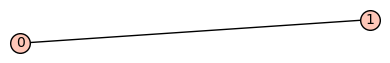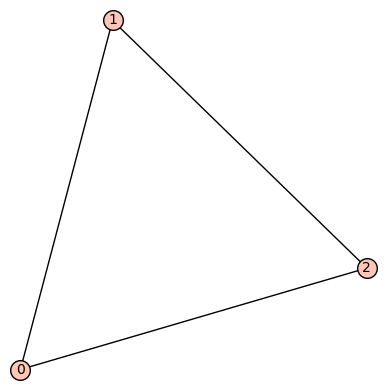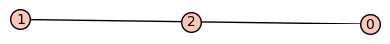# Revision history [back]

My understanding is that, since you are looking for digraphs up to edge-orientation, and since every undirected graph can be oriented in an acyclic way (just define a linear order on the vertices the vertices and orient the edges accordingly), you are looking for connected undirected graphs up to isomorphism. Sage does that easily:

For example, imagine that you want to plot each of them, you can do:

sage: n = 2
sage: for G in graphs.nauty_geng('{} -c'.format(n)):
....:     show(G)


This will result as a single graph:If you set n=3, you will get:and so on.

My understanding is that, since you are looking for digraphs up to edge-orientation, and since every undirected graph can be oriented in an acyclic way (just define a linear order on the vertices the vertices and orient the edges accordingly), you are looking for connected undirected graphs up to isomorphism. Sage does that easily:

For example, imagine that you want to plot each of them, you can do:

sage: n = 2
sage: for G in graphs.nauty_geng('{} -c'.format(n)):
....:     show(G)


This will result as a single graph:If you set n=3, you will get:and so on.

Regarding your string representation, i am not sure how you plan to pass it to gap, but you can easily enumerate the edges of a graph G as follows:

sage: [[a,b,"a_{}".format(i)] for (i,(a,b)) in enumerate(G.edges(labels=False))]
[[0, 1, 'a_0'], [0, 2, 'a_1'], [1, 2, 'a_2']]